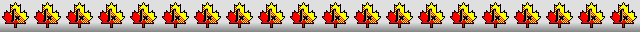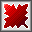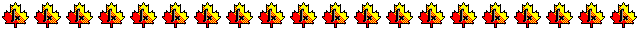Maple worksheets on the derivation of order 12 Runge-Kutta schemesDerivation of 25 stage, order 12 Runge-Kutta schemes - RKcoeff12a.mws   RKcoeff12b.mws   RKcoeff12c.mws   RKcoeff12d.mws

Derivation of embedded order 9 schemes for order 12 Runge-Kutta methods - RKcoeff12a_(9).mws   RKcoeff12c_(9).mws   RKcoeff12d_(9).mws

Derivation of embedded order 10 and 11 schemes for an order 12 Runge-Kutta method - RKcoeff12a_(10,11).mws

• Defective embedded order 10 and 11 schemes for Terry Feagin's order 12 scheme

Derivation of 25 stage, order 12 Runge-Kutta schemes - RKcoeff12e.mws   RKcoeff12f.mws   RKcoeff12g.mws   RKcoeff12h.mws

• Hiroshi Ono's scheme and some modifications of his scheme.
See: "On the 25 stage 12th order explicit Runge-Kutta method", by Hiroshi Ono.
Transactions of the Japan Society for Industrial and Applied Mathematics, Vol. 6, No. 3, (2006) pages 177 to 186.

• Coefficients for the schemes and some properties
RKcoeff12e_1.pdf     RKcoeff12f_1.pdf     RKcoeff12g_1.pdf     RKcoeff12h_1.pdf

Derivation of an embedded order 9 scheme for an order 12 Runge-Kutta method - RKcoeff12h_(9).mws

• An embedded order 9 scheme for a modification Hiroshi Ono's scheme
A summary of the method of construction of the combined scheme is given in the pdf file - RKcoeff12(9).pdf

• Coefficients for the combined scheme and some properties
RKcoeff12h(9)_1.pdf

Derivation of 31 stage, combined order 11 and 12 Runge-Kutta schemes - RKcoeff12k.mws    RKcoeff12m.mws    RKcoeff12n.mws

Procedures related to the construction of Runge-Kutta schemes - butcher.zip

Procedures for root-finding and determining minimum points  - roots.zip

Procedures for numerical integration  - intg.zip

Top of page

Main index